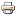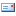# What is the strength of materialsThe tensile strength is a material parameter that is not only important in the case of a tensile load. It indicates how strong a material can be subjected to. If the tensile strength value is exceeded, the material fails. It should be noted that the material deforms plastically (i.e. permanently) before it reaches its tensile strength.
The minimum value or the guaranteed value of the tensile strength is relevant for the mechanical design of components and constructions. This value is used for the strength calculation (in addition to other relevant values, of course).

Tensile strenght:
- Formula symbol (for metals): Rm
- SI unit: MPa (Megapascal) (= N / mm²)

The tensile strength is in N / mm2 (Force per area) and is therefore a voltage. This means that we are looking at a force that is evenly distributed over the cross-section of a body (component).
The tensile strength indicates the force at which a workpiece with a certain cross-section and consisting of a certain material will fail when it is subjected to tensile stress.### Example - steel S235JR

To illustrate the term tensile strength and what it means, here is an example:

We take a piece of steel as a test specimen in order to determine its tensile strength in a tensile test. Since the tensile strength is material-specific, it is important to know which material the sample is made of. In our case, the sample should consist of the structural steel S235JR (formerly St37-2).
According to the material data, the steel S235JR has a minimum tensile strength of 370 N / mm2. The sample should therefore fail at the earliest at a tensile load of 370 N (or 37.7 kg) per millimeter of cross-sectional area.

As already written further above, a permanent deformation occurs even before the failure of the specimen. In the mechanical design of components and structures, irreversible deformation is generally not permitted. For this reason, it is primarily not the minimum tensile strength of the material that is calculated, but its minimum yield strength (R.e).

Yield point or yield point
- Formula symbol (for metals): Rp or Re
- SI unit: MPa (Megapascal) (= N / mm²)

Tensile strength and yield point are determined specifically for each material in a so-called tensile test.